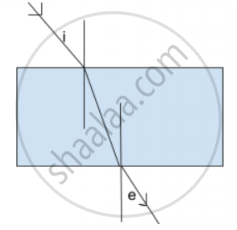# In the following diagram if ∠ i = 40°, then ∠ e = _______? - Science and Technology 1

MCQ
Fill in the Blanks

In the following diagram if ∠ i = 40°, then ∠ e = _______?• 50°

• 40°

• 60°

• 90°

#### Solution

In the following diagram if ∠ i = 40°, then ∠ e = 40°.

Concept: Law of Refraction of Light
Is there an error in this question or solution?

Share